# Lecture 1

## Knowledge

Humans reason based on existing knowledge and draw conclusions. The concept of representing knowledge and drawing conclusions from it is also used in AI, and in this lecture we will explore how we can achieve this behavior.

Knowledge-Based Agents

These are agents that reason by operating on internal representations of knowledge.

What does “reasoning based on knowledge to draw a conclusion” mean?

Let’s start answering this with a Harry Potter example. Consider the following sentences:

1. If it didn’t rain, Harry visited Hagrid today.
2. Harry visited Hagrid or Dumbledore today, but not both.
3. Harry visited Dumbledore today.

Based on these three sentences, we can answer the question “did it rain today?”, even though none of the individual sentences tells us anything about whether it is raining today. Here is how we can go about it: looking at sentence 3, we know that Harry visited Dumbledore. Looking at sentence 2, we know that Harry visited either Dumbledore or Hagrid, and thus we can conclude

1. Harry did not visit Hagrid.

Now, looking at sentence 1, we understand that if it didn’t rain, Harry would have visited Hagrid. However, knowing sentence 4, we know that this is not the case. Therefore, we can conclude

1. It rained today.

To come to this conclusion, we used logic, and today’s lecture explores how AI can use logic to reach to new conclusions based on existing information.

Sentence

A sentence is an assertion about the world in a knowledge representation language. A sentence is how AI stores knowledge and uses it to infer new information.

## Propositional Logic

Propositional logic is based on propositions, statements about the world that can be either true or false, as in sentences 1-5 above.

Propositional Symbols

Propositional symbols are most often letters (P, Q, R) that are used to represent a proposition.

Logical Connectives

Logical connectives are logical symbols that connect propositional symbols in order to reason in a more complex way about the world.

• Not (¬) inverses the truth value of the proposition. So, for example, if P: “It is raining,” then ¬P: “It is not raining”.

Truth tables are used to compare all possible truth assignments to propositions. This tool will help us better understand the truth values of propositions when connected with different logical connectives. For example, below is our first truth table:

P ¬P
false true
true false
• And (∧) connects two different propositions. When these two proposition, P and Q, are connected by ∧, the resulting proposition P ∧ Q is true only in the case that both P and Q are true.

P Q P ∧ Q
false false false
false true false
true false false
true true true
• Or (∨) is true as as long as either of its arguments is true. This means that for P ∨ Q to be true, at least one of P or Q has to be true.

P Q P ∨ Q
false false false
false true true
true false true
true true true

It is worthwhile to mention that there are two types of Or: an inclusive Or and an exclusive Or. In an exclusive Or, P ∨ Q is false if P ∧ Q is true. That is, an exclusive Or requires only one of its arguments to be true and not both. An inclusive Or is true if any of P, Q, or P ∧ Q is true. In the case of Or (∨), the intention is an inclusive Or.

A couple of side notes not mentioned in lecture:

• Sometimes an example helps understand inclusive versus exclusive Or. Inclusive Or: “in order to eat dessert, you have to clean your room or mow the lawn.” In this case, if you do both chores, you will still get the cookies. Exclusive Or: “For dessert, you can have either cookies or ice cream.” In this case, you can’t have both.
• If you are curious, the exclusive Or is often shortened to XOR and a common symbol for it is ⊕).
• Implication (→) represents a structure of “if P then Q.” For example, if P: “It is raining” and Q: “I’m indoors”, then P → Q means “If it is raining, then I’m indoors.” In the case of P implies Q (P → Q), P is called the antecedent and Q is called the consequent.

When the antecedent is true, the whole implication is true in the case that the consequent is true (that makes sense: if it is raining and I’m indoors, then the sentence “if it is raining, then I’m indoors” is true). When the antecedent is true, the implication is false if the consequent is false (if I’m outside while it is raining, then the sentence “If it is raining, then I’m indoors” is false). However, when the antecedent is false, the implication is always true, regardless of the consequent. This can sometimes be a confusing concept. Logically, we can’t learn anything from an implication (P → Q) if the antecedent (P) is false. Looking at our example, if it is not raining, the implication doesn’t say anything about whether I’m indoors or not. I could be an indoors type and never walk outside, even when it is not raining, or I could be an outdoors type and be outside all the time when it is not raining. When the antecedent is false, we say that the implication is trivially true.

P Q P → Q
false false true
false true true
true false false
true true true
• Biconditional (↔) is an implication that goes both directions. You can read it as “if and only if.” P ↔ Q is the same as P → Q and Q → P taken together. For example, if P: “It is raining.” and Q: “I’m indoors,” then P ↔ Q means that “If it is raining, then I’m indoors,” and “if I’m indoors, then it is raining.” This means that we can infer more than we could with a simple implication. If P is false, then Q is also false; if it is not raining, we know that I’m also not indoors.

P Q P ↔ Q
false false true
false true false
true false false
true true true

Model

The model is an assignment of a truth value to every proposition. To reiterate, propositions are statements about the world that can be either true or false. However, knowledge about the world is represented in the truth values of these propositions. The model is the truth-value assignment that provides information about the world.

For example, if P: “It is raining.” and Q: “It is Tuesday.”, a model could be the following truth-value assignment: {P = True, Q = False}. This model means that it is raining, but it is not Tuesday. However, there are more possible models in this situation (for example, {P = True, Q = True}, where it is both raining an a Tuesday). In fact, the number of possible models is 2 to the power of the number of propositions. In this case, we had 2 propositions, so 2²=4 possible models.

Knowledge Base (KB)

The knowledge base is a set of sentences known by a knowledge-based agent. This is knowledge that the AI is provided about the world in the form of propositional logic sentences that can be used to make additional inferences about the world.

Entailment (⊨)

If α ⊨ β (α entails β), then in any world where α is true, β is true, too.

For example, if α: “It is a Tuesday in January” and β: “It is a Tuesday,” then we know that α ⊨ β. If it is true that it it a Tuesday in January, we also know that it is a Tuesday. Entailment is different from implication. Implication is a logical connective between two propositions. Entailment, on the other hand, is a relation that means that if all the information in α is true, then all the information in β is true.

## Inference

Inference is the process of deriving new sentences from old ones.

For instance, in the Harry Potter example earlier, sentences 4 and 5 were inferred from sentences 1, 2, and 3.

There are multiple ways to infer new knowledge based on existing knowledge. First, we will consider the Model Checking algorithm.

• To determine if KB ⊨ α (in other words, answering the question: “can we conclude that α is true based on our knowledge base”)
• Enumerate all possible models.
• If in every model where KB is true, α is true as well, then KB entails α (KB ⊨ α).

Consider the following example:

P: It is a Tuesday. Q: It is raining. R: Harry will go for a run. KB: (P ∧ ¬Q) → R (in words, P and not Q imply R) P (P is true) ¬Q (Q is false) Query: R (We want to know whether R is true or false; Does KB ⊨ R?)

To answer the query using the Model Checking algorithm, we enumerate all possible models.

P Q R KB
false false false
false false true
false true false
false true true
true false false
true false true
true true false
true true true

Then, we go through every model and check whether it is true given our Knowledge Base.

First, in our KB, we know that P is true. Thus, we can say that the KB is false in all models where P is not true.

P Q R KB
false false false false
false false true false
false true false false
false true true false
true false false
true false true
true true false
true true true

Next, similarly, in our KB, we know that Q is false. Thus, we can say that the KB is false in all models where Q is true.

P Q R KB
false false false false
false false true false
false true false false
false true true false
true false false
true false true
true true false false
true true true false

Finally, we are left with two models. In both, P is true and Q is false. In one model R is true and in the other R is false. Due to (P ∧ ¬Q) → R being in our KB, we know that in the case where P is true and Q is false, R must be true. Thus, we say that our KB is false for the model where R is false, and true for the model where R is true.

P Q R KB
false false false false
false false true false
false true false false
false true true false
true false false false
true false true true
true true false false
true true true false

Looking at this table, there is only one model where our knowledge base is true. In this model, we see that R is also true. By our definition of entailment, if R is true in all models where the KB is true, then KB ⊨ R.

Next, let’s look at how knowledge and logic can be represented as code.

from logic import *

# Create new classes, each having a name, or a symbol, representing each proposition.
rain = Symbol("rain")  # It is raining.
hagrid = Symbol("hagrid")  # Harry visited Hagrid
dumbledore = Symbol("dumbledore")  # Harry visited Dumbledore

# Save sentences into the KB
knowledge = And(  # Starting from the "And" logical connective, becasue each proposition represents knowledge that we know to be true.

Implication(Not(rain), hagrid),  # ¬(It is raining) → (Harry visited Hagrid)

Or(hagrid, dumbledore),  # (Harry visited Hagrid) ∨ (Harry visited Dumbledore).

Not(And(hagrid, dumbledore)),  # ¬(Harry visited Hagrid ∧ Harry visited Dumbledore) i.e. Harry did not visit both Hagrid and Dumbledore.

dumbledore  # Harry visited Dumbledore. Note that while previous propositions contained multiple symbols with connectors, this is a proposition consisting of one symbol. This means that we take as a fact that, in this KB, Harry visited Dumbledore.
)


To run the Model Checking algorithm, the following information is needed:

• Knowledge Base, which will be used to draw inferences
• A query, or the proposition that we are interested in whether it is entailed by the KB
• Symbols, a list of all the symbols (or atomic propositions) used (in our case, these are rain, hagrid, and dumbledore)
• Model, an assignment of truth and false values to symbols

The model checking algorithm looks as follows:

def check_all(knowledge, query, symbols, model):

# If model has an assignment for each symbol
# (The logic below might be a little confusing: we start with a list of symbols. The function is recursive, and every time it calls itself it pops one symbol from the symbols list and generates models from it. Thus, when the symbols list is empty, we know that we finished generating models with every possible truth assignment of symbols.)
if not symbols:

# If knowledge base is true in model, then query must also be true
if knowledge.evaluate(model):
return query.evaluate(model)
return True
else:

# Choose one of the remaining unused symbols
remaining = symbols.copy()
p = remaining.pop()

# Create a model where the symbol is true
model_true = model.copy()
model_true[p] = True

# Create a model where the symbol is false
model_false = model.copy()
model_false[p] = False

# Ensure entailment holds in both models
return(check_all(knowledge, query, remaining, model_true) and check_all(knowledge, query, remaining, model_false))


Note that we are interested only in the models where the KB is true. If the KB is false, then the conditions that we know to be true are not occurring in these models, making them irrelevant to our case.

An example from outside lecture: Let P: Harry plays seeker, Q: Oliver plays keeper, R: Gryffindor wins. Our KB specifies that P Q (P ∧ Q) → R. In other words, we know that P is true, i.e. Harry plays seeker, and that Q is true, i.e. Oliver plays keeper, and that if both P and Q are true, then R is true, too, meaning that Gryffindor wins the match. Now imagine a model where Harry played beater instead of seeker (thus, Harry did not play seeker, ¬P). Well, in this case, we don’t care whether Gryffindor won (whether R is true or not), because we have the information in our KB that Harry played seeker and not beater. We are only interested in the models where, as in our case, P and Q are true.)

Further, the way the check_all function works is recursive. That is, it picks one symbol, creates two models, in one of which the symbol is true and in the other the symbol is false, and then calls itself again, now with two models that differ by the truth assignment of this symbol. The function will keep doing so until all symbols will have been assigned truth-values in the models, leaving the list symbols empty. Once it is empty (as identified by the line if not symbols), in each instance of the function (wherein each instance holds a different model), the function checks whether the KB is true given the model. If the KB is true in this model, the function checks whether the query is true, as described earlier.

## Knowledge Engineering

Knowledge engineering is the process of figuring out how to represent propositions and logic in AI.

Let’s practice knowledge engineering using the game Clue.

In the game, a murder was committed by a person, using a tool in a location. People, tools, and locations are represented by cards. One card of each category is picked at random and put in an envelope, and it is up to the participants to uncover whodunnit. Participants do so by uncovering cards and deducing from these clues what must be in the envelope. We will use the Model Checking algorithm from before to uncover the mystery. In our model, we mark as True items that we know are related to the murder and False otherwise.

For our purposes, suppose we have three people: Mustard, Plum, and Scarlet, three tools: knife, revolver, and wrench, and three locations: ballroom, kitchen, and library.

We can start creating our knowledge base by adding the rules of the game. We know for certain that one person is the murderer, that one tool was used, and that the murder happened in one location. This can be represented in propositional logic the following way:

(Mustard ∨ Plum ∨ Scarlet)

(knife ∨ revolver ∨ wrench)

(ballroom ∨ kitchen ∨ library)

The game starts with each player seeing one person, one tool, and one location, thus knowing that they are not related to the murder. Players do not share the information that the saw in these cards. Suppose our player gets the cards of Mustard, kitchen, and revolver. Thus, we know that these are not related to the murder and we can add to our KB

¬(Mustard)

¬(kitchen)

¬(revolver)

In other situations in the game, one can make a guess, suggesting one combination of person, tool and location. Suppose that the guess is that Scarlet used a wrench to commit the crime in the library. If this guess is wrong, then the following can be deduced and added to the KB:

(¬Scarlet ∨ ¬library ∨ ¬wrench)

Now, suppose someone shows us the Plum card. Thus, we can add

¬(Plum)

to our KB.

At this point, we can conclude that the murderer is Scarlet, since it has to be one of Mustard, Plum, and Scarlet, and we have evidence that the first two are not it.

Adding just one more piece of knowledge, for example, that it is not the ballroom, can give us more information. First, we update our KB

¬(ballroom)

And now, using multiple previous pieces of data, we can deduce that Scarlet committed the murder with a knife in the library. We can deduce that it’s the library because it has to be either the ballroom, the kitchen, or the library, and the first two were proven to not be the locations. However, when someone guessed Scarlet, library, wrench, the guess was false. Thus, at least one of the elements in this statement has to be false. Since we know both Scarlet and library to be true, we know that the wrench is the false part here. Since one of the three instruments has to be true, and it’s not the wrench nor the revolver, we can conclude that it is the knife.

Here is how the information would be added to the knowledge base in Python:

# Add the clues to the KB
knowledge = And(

# Start with the game conditions: one item in each of the three categories has to be true.
Or(mustard, plum, scarlet),
Or(ballroom, kitchen, library),
Or(knife, revolver, wrench),

# Add the information from the three initial cards we saw
Not(mustard),
Not(kitchen),
Not(revolver),

# Add the guess someone made that it is Scarlet, who used a wrench in the library
Or(Not(scarlet), Not(library), Not(wrench)),

# Add the cards that we were exposed to
Not(plum),
Not(ballroom)
)


We can look at other logic puzzles as well. Consider the following example: four different people, Gilderoy, Pomona, Minerva, and Horace, are assigned to four different houses, Gryffindor, Hufflepuff, Ravenclaw, and Slytherin. There is exactly one person in each house. Representing the puzzle’s conditions in propositional logic is quite cumbersome. First, each of the possible assignments will have to be a proposition in itself: MinervaGryffindor, MinervaHufflepuff, MinervaRavenclaw, MinervaSlytherin, PomonaGryffindor… Second, to represent that each person belongs to a house, an Or statement is required with all the possible house assignments per person

(MinervaGryffindor ∨ MinervaHufflepuff ∨ MinervaRavenclaw ∨ MinervaSlytherin), repeat for every person.

Then, to encode that if one person is assigned to one house, they are not assigned to the other houses, we will write

(MinervaGryffindor → ¬MinervaHufflepuff) ∧ (MinervaGryffindor → ¬MinervaRavenclaw) ∧ (MinervaGryffindor → ¬MinervaSlytherin) ∧ (MinervaHufflepuff → ¬MinervaGryffindor)…

and so on for all houses and all people. A solution to this inefficiency is offered in the section on first order logic. However, this type of riddle can still be solved with either type of logic, given enough cues.

Another type of puzzle that can be solved using propositional logic is a Mastermind game. In this game, player one arranges colors in a certain order, and then player two has to guess this order. Each turn, player two makes a guess, and player one gives back a number, indicating how many colors player two got right. Let’s simulate a game with four colors. Suppose player two suggests the following ordering:Player one answers “two.” Thus we know that some two of the colors are in the correct position, and the other two are in the wrong place. Based on this information, player two tries to switch the locations of two colors.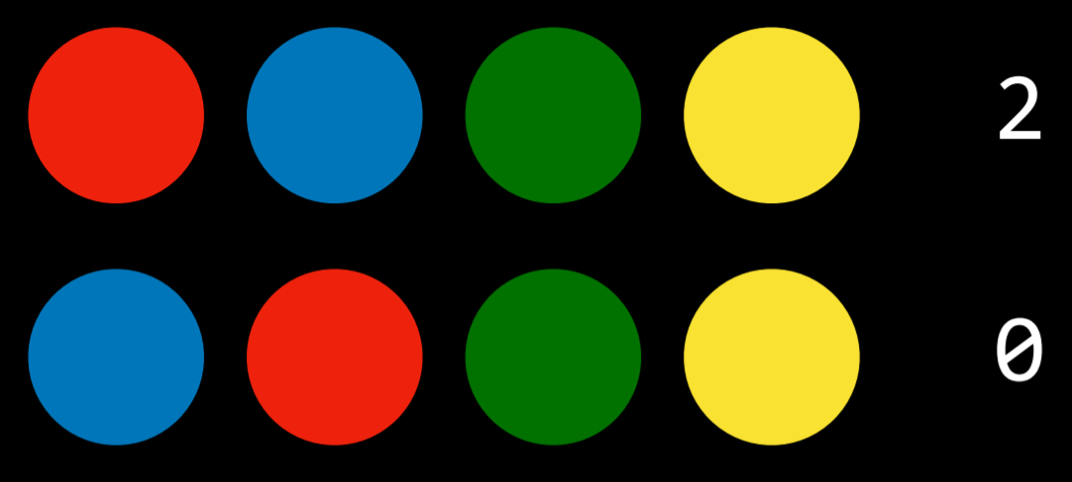Now player one answers “zero.” Thus, player two knows that the switched colors were in the right location initially, which means the untouched two colors were in the wrong location. Player two switches them.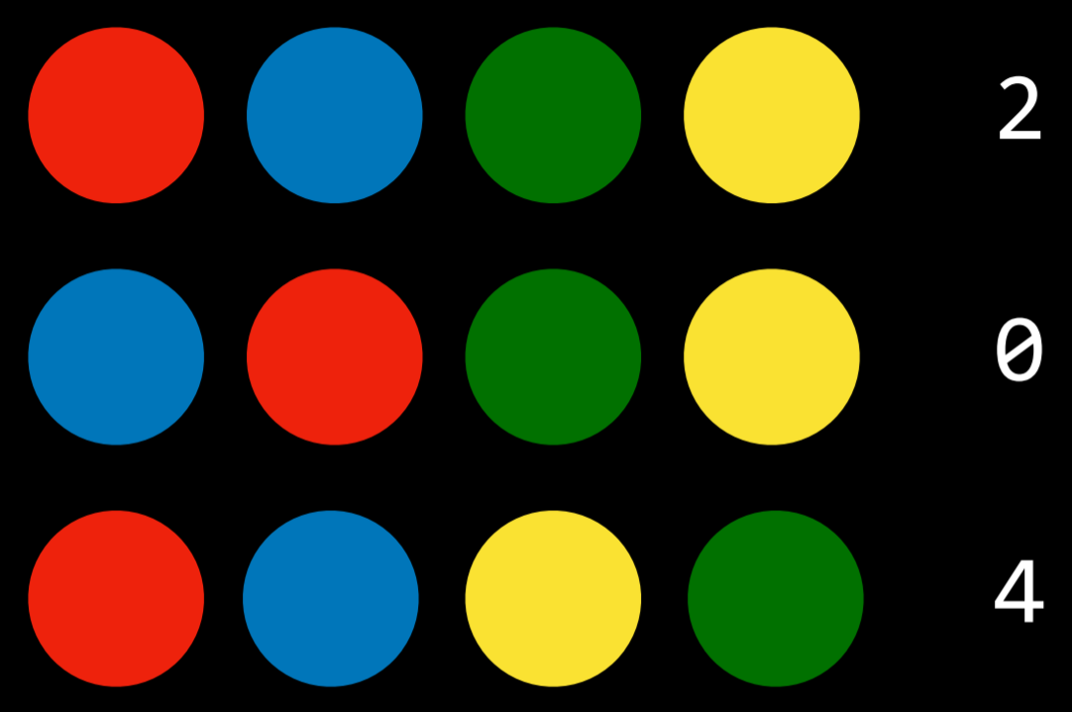Player one says “four” and the game is over.

Representing this in propositional logic would require us to have (number of colors)² atomic propositions. So, in the case of four colors, we would have the propositions red0, red1, red2, red3, blue0… standing for color and position. The next step would be representing the rules of the game in propositional logic (that there is only one color in each position and no colors repeat) and adding them to the KB. The final step would be adding all the cues that we have to the KB. In our case, we would add that, in the first guess, two positions were wrong and two were right, and in the second guess, none was right. Using this knowledge, a Model Checking algorithm can give us the solution to the puzzle.

## Inference Rules

Model Checking is not an efficient algorithm because it has to consider every possible model before giving the answer (a reminder: a query R is true if under all the models (truth assignments) where the KB is true, R is true as well). Inference rules allow us to generate new information based on existing knowledge without considering every possible model.

Inference rules are usually represented using a horizontal bar that separates the top part, the premise, from the bottom part, the conclusion. The premise is whatever knowledge we have, and the conclusion is what knowledge can be generated based on the premise.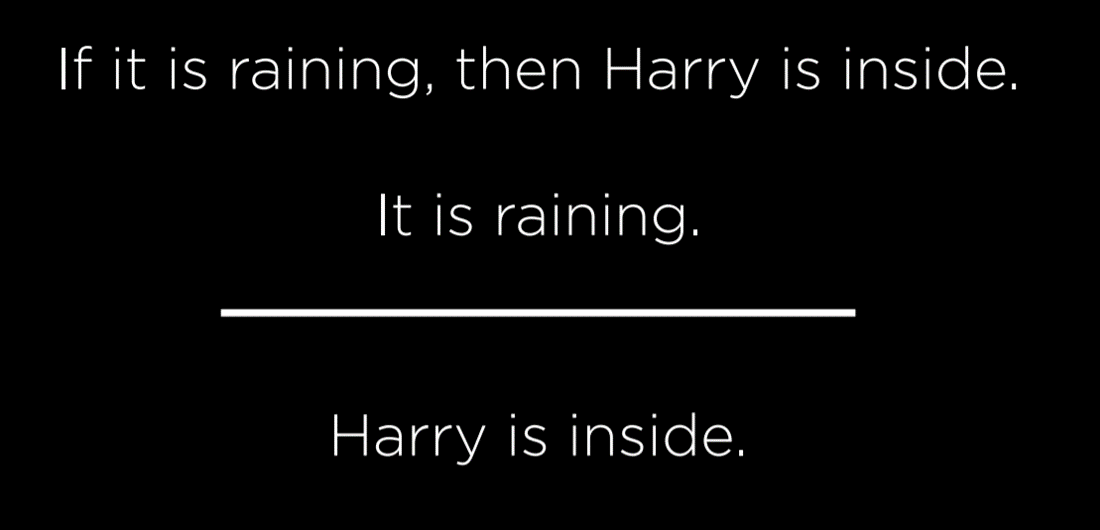In this example, our premise consists of the following propositions:

• If it is raining, then Harry is inside.
• It is raining.

Based on this, most reasonable humans can conclude that

• Harry is inside.

Modus Ponens

The type of inference rule we use in this example is Modus Ponens, which is a fancy way of saying that if we know an implication and its antecedent to be true, then the consequent is true as well.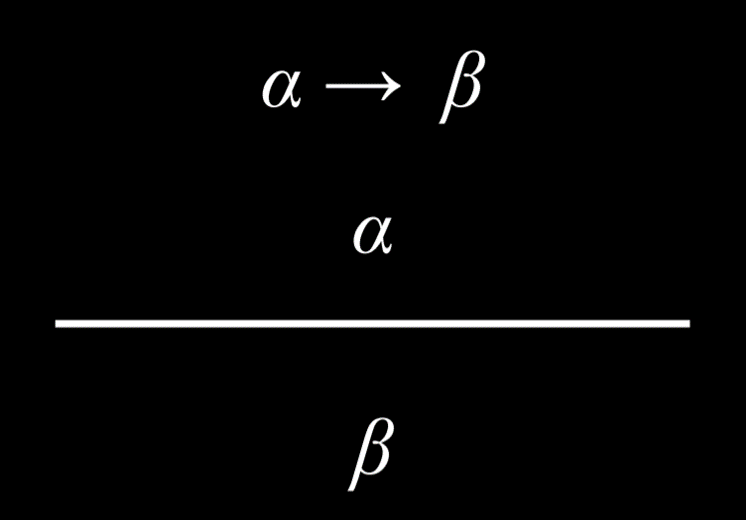And Elimination

If an And proposition is true, then any one atomic proposition within it is true as well. For example, if we know that Harry is friends with Ron and Hermione, we can conclude that Harry is friends with Hermione.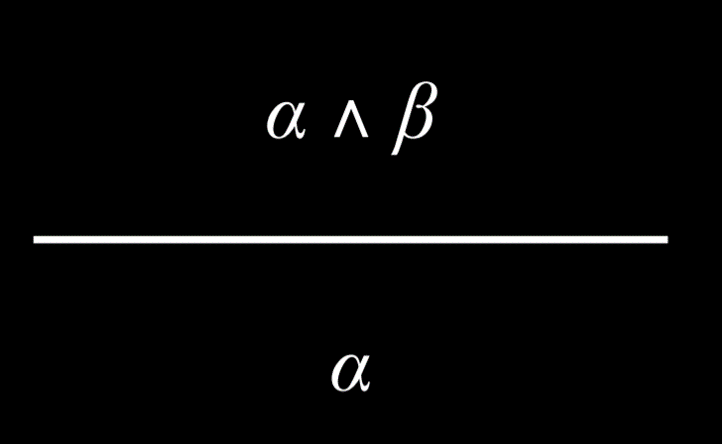Double Negation Elimination

A proposition that is negated twice is true. For example, consider the proposition “It is not true that Harry did not pass the test”. We can parse it the following way: “It is not true that (Harry did not pass the test)”, or “¬(Harry did not pass the test)”, and, finally “¬(¬(Harry passed the test)).” The two negations cancel each other, marking the proposition “Harry passed the test” as true.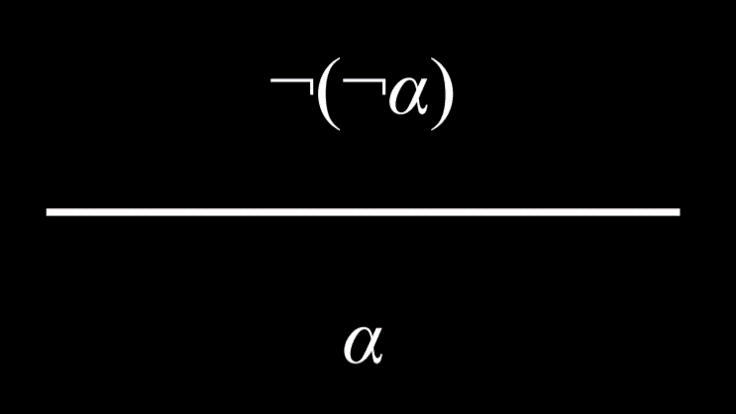Implication Elimination

An implication is equivalent to an Or relation between the negated antecedent and the consequent. As an example, the proposition “If it is raining, Harry is inside” is equivalent to the proposition “(it is not raining) or (Harry is inside).”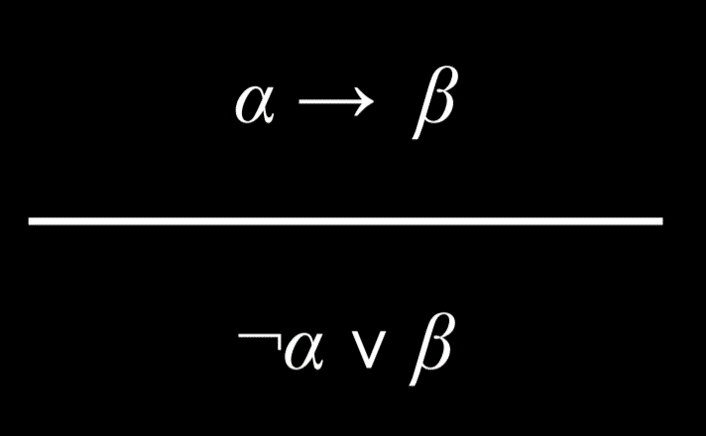This one can be a little confusing. However, consider the following truth table:

P Q P → Q ¬P ∨ Q
false false true true
false true true true
true false false false
true true true true

Since P → Q and ¬P ∨ Q have the same truth-value assignment, we know them to be equivalent logically. Another way to think about this is that an implication is true if either of two possible conditions is met: first, if the antecedent is false, the implication is trivially true (as discussed earlier, in the section on implication). This is represented by the negated antecedent P in ¬P ∨ Q, meaning that the proposition is always true if P is false. Second, the implication is true when the antecedent is true only when the consequent is true as well. That is, if P and Q are both true, then ¬P ∨ Q is true. However, if P is true and Q is not, then ¬P ∨ Q is false.

Biconditional Elimination

A biconditional proposition is equivalent to an implication and its inverse with an And connective. For example, “It is raining if and only if Harry is inside” is equivalent to (“If it is raining, Harry is inside” And “If Harry is inside, it is raining”).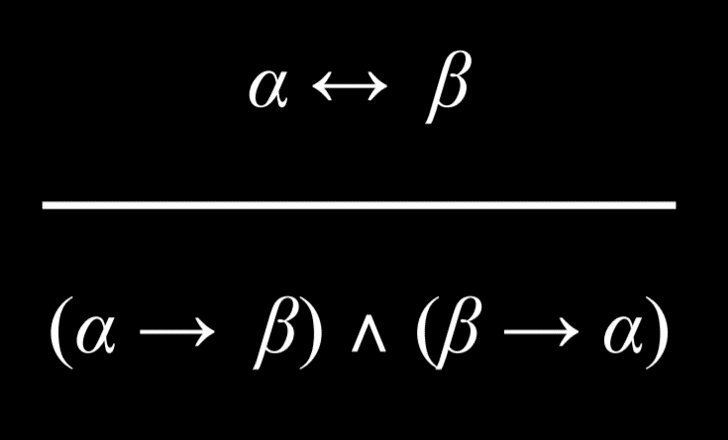De Morgan’s Law

It is possible to turn an And connective into an Or connective. Consider the following proposition: “It is not true that both Harry and Ron passed the test.” From this, it is possible to conclude that “It is not true that Harry passed the test” Or “It is not true that Ron passed the test.” That is, for the And proposition earlier to be true, at least one of the propositions in the Or propositions must be true.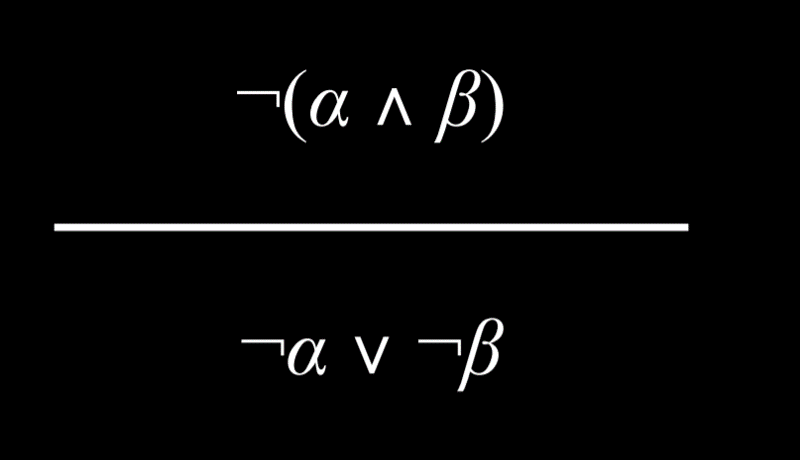Similarly, it is possible to conclude the reverse. Consider the proposition “It is not true that Harry or Ron passed the test.” This can be rephrased as “Harry did not pass the test” And “Ron did not pass the test.”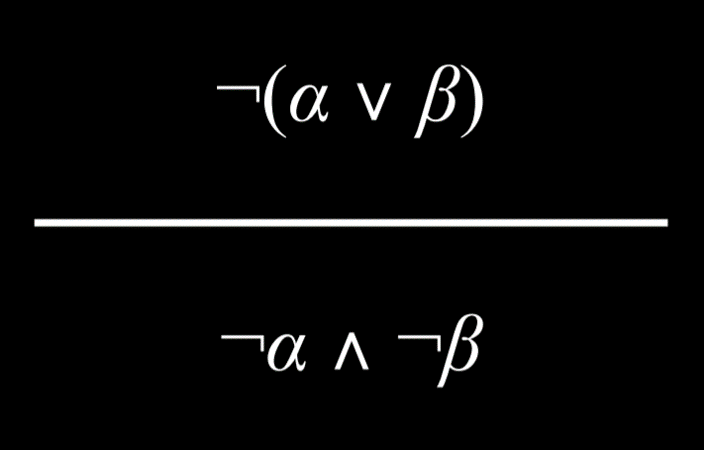Distributive Property

A proposition with two elements that are grouped with And or Or connectives can be distributed, or broken down into, smaller units consisting of And and Or.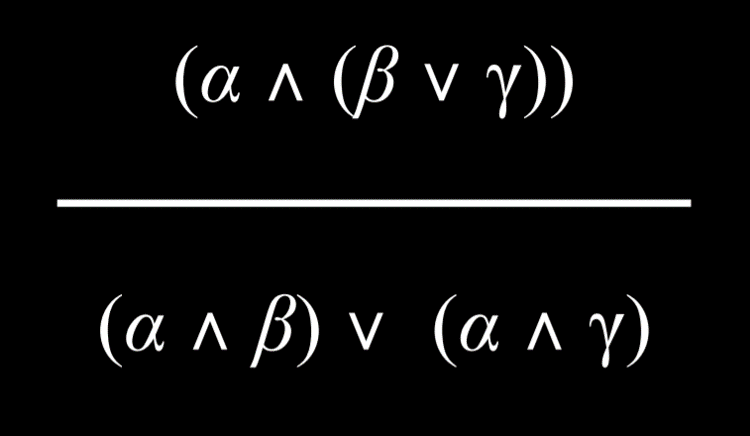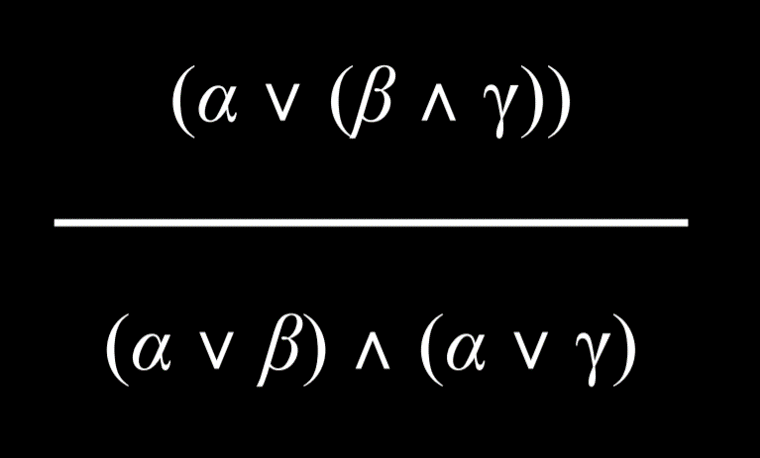Knowledge and Search Problems

Inference can be viewed as a search problem with the following properties:

• Initial state: starting knowledge base
• Actions: inference rules
• Transition model: new knowledge base after inference
• Goal test: checking whether the statement that we are trying to prove is in the KB
• Path cost function: the number of steps in the proof

This shows just how versatile search algorithms are, allowing us to derive new information based on existing knowledge using inference rules.

## Resolution

Resolution is a powerful inference rule that states that if one of two atomic propositions in an Or proposition is false, the other has to be true. For example, given the proposition “Ron is in the Great Hall” Or “Hermione is in the library”, in addition to the proposition “Ron is not in the Great Hall,” we can conclude that “Hermione is in the library.” More formally, we can define resolution the following way: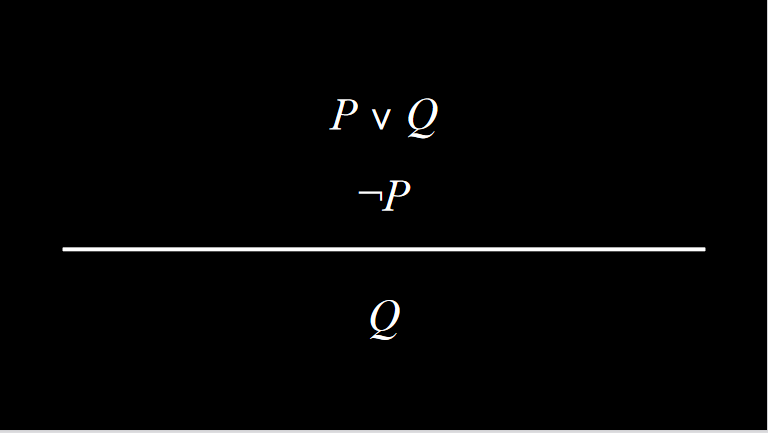Resolution relies on Complementary Literals, two of the same atomic propositions where one is negated and the other is not, such as P and ¬P.

Resolution can be further generalized. Suppose that in addition to the proposition “Ron is in the Great Hall” Or “Hermione is in the library”, we also know that “Ron is not in the Great Hall” Or “Harry is sleeping.” We can infer from this, using resolution, that “Hermione is in the library” Or “Harry is sleeping.” To put it in formal terms: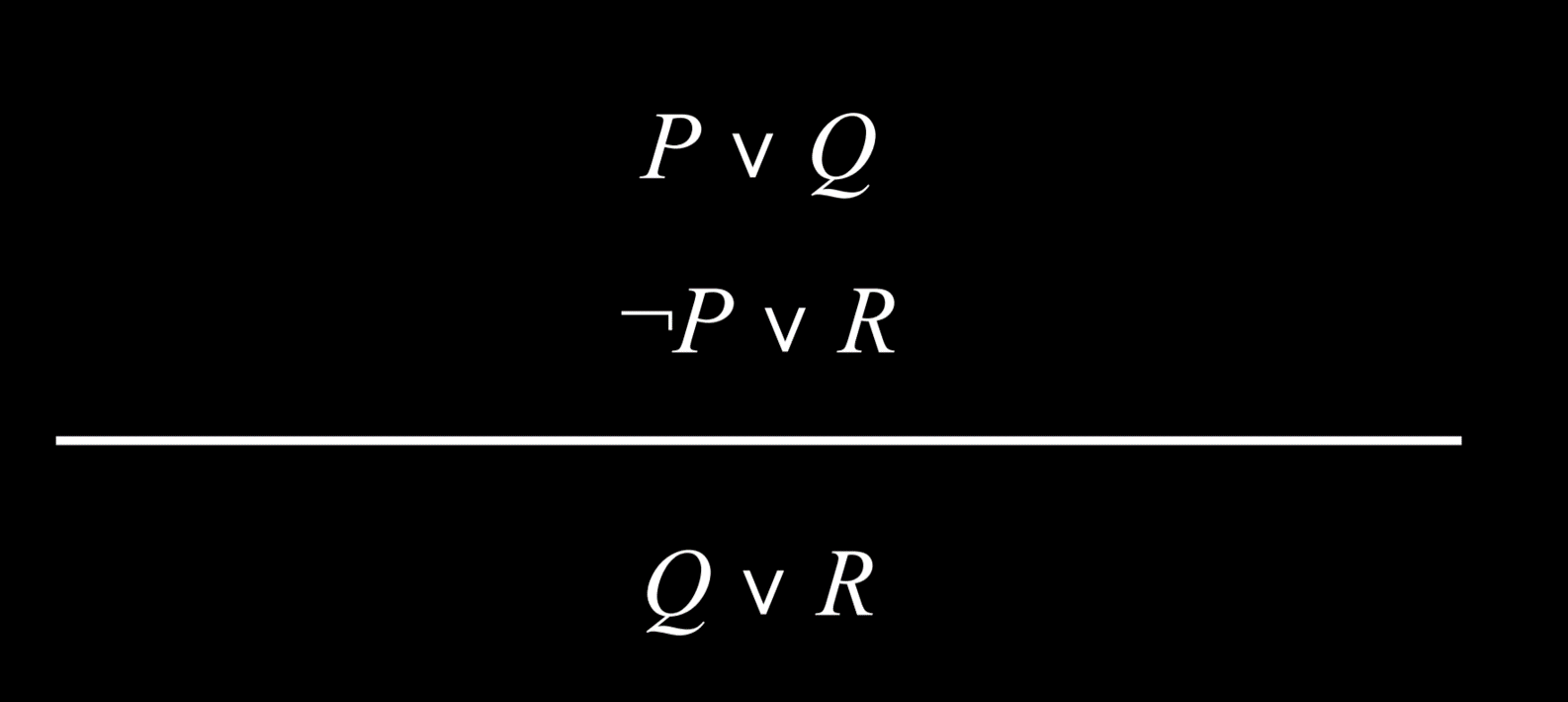Complementary literals allow us to generate new sentences through inferences by resolution. Thus, inference algorithms locate complementary literals to generate new knowledge.

A Clause is a disjunction of literals (a propositional symbol or a negation of a propositional symbol, such as P, ¬P). A disjunction consists of propositions that are connected with an Or logical connective (P ∨ Q ∨ R). A conjunction, on the other hand, consists of propositions that are connected with an And logical connective (P ∧ Q ∧ R). Clauses allow us to convert any logical statement into a Conjunctive Normal Form (CNF), which is a conjunction of clauses, for example: (A ∨ B ∨ C) ∧ (D ∨ ¬E) ∧ (F ∨ G).

Steps in Conversion of Propositions to Conjunctive Normal Form

• Eliminate biconditionals
• Turn (α ↔ β) into (α → β) ∧ (β → α).
• Eliminate implications
• Turn (α → β) into ¬α ∨ β.
• Move negation inwards until only literals are being negated (and not clauses), using De Morgan’s Laws.
• Turn ¬(α ∧ β) into ¬α ∨ ¬β

Here’s an example of converting (P ∨ Q) → R to Conjunctive Normal Form:

• (P ∨ Q) → R
• ¬(P ∨ Q) ∨ R /Eliminate implication
• (¬P ∧ ¬Q) ∨ R /De Morgan’s Law
• (¬P ∨ R) ∧ (¬Q ∨ R) /Distributive Law

At this point, we can run an inference algorithm on the conjunctive normal form. Occasionally, through the process of inference by resolution, we might end up in cases where a clause contains the same literal twice. In these cases, a process called factoring is used, where the duplicate literal is removed. For example, (P ∨ Q ∨ S) ∧ (¬P ∨ R ∨ S) allow us to infer by resolution that (Q ∨ S ∨ R ∨ S). The duplicate S can be removed to give us (Q ∨ R ∨ S).

Resolving a literal and its negation, i.e. ¬P and P, gives the empty clause (). The empty clause is always false, and this makes sense because it is impossible that both P and ¬P are true. This fact is used by the resolution algorithm.

• To determine if KB ⊨ α:
• Check: is (KB ∧ ¬α) a contradiction?
• If so, then KB ⊨ α.
• Otherwise, no entailment.

Proof by contradiction is a tool used often in computer science. If our knowledge base is true, and it contradicts ¬α, it means that ¬α is false, and, therefore, α must be true. More technically, the algorithm would perform the following actions:

• To determine if KB ⊨ α:
• Convert (KB ∧ ¬α) to Conjunctive Normal Form.
• Keep checking to see if we can use resolution to produce a new clause.
• If we ever produce the empty clause (equivalent to False), congratulations! We have arrived at a contradiction, thus proving that KB ⊨ α.
• However, if contradiction is not achieved and no more clauses can be inferred, there is no entailment.

Here is an example that illustrates how this algorithm might work:

• Does (A ∨ B) ∧ (¬B ∨ C) ∧ (¬C) entail A?
• First, to prove by contradiction, we assume that A is false. Thus, we arrive at (A ∨ B) ∧ (¬B ∨ C) ∧ (¬C) ∧ (¬A).
• Now, we can start generating new information. Since we know that C is false (¬C), the only way (¬B ∨ C) can be true is if B is false, too. Thus, we can add (¬B) to our KB.
• Next, since we know (¬B), the only way (A ∨ B) can be true is if A is true. Thus, we can add (A) to our KB.
• Now our KB has two complementary literals, (A) and (¬A). We resolve them, arriving at the empty set, (). The empty set is false by definition, so we have arrived at a contradiction.

## First Order Logic

First order logic is another type of logic that allows us to express more complex ideas more succinctly than propositional logic. First order logic uses two types of symbols: Constant Symbols and Predicate Symbols. Constant symbols represent objects, while predicate symbols are like relations or functions that take an argument and return a true or false value.

For example, we return to the logic puzzle with different people and house assignments at Hogwarts. The constant symbols are people or houses, like Minerva, Pomona, Gryffindor, Hufflepuff, etc. The predicate symbols are properties that hold true or false of some constant symbols. For example, we can express the idea that Minerva is a person using the sentence Person(Minerva). Similarly, we can express the idea the Gryffindor is a house using the sentence House(Gryffindor). All the logical connectives work in first order logic the same way as before. For example, ¬House(Minerva) expresses the idea that Minerva is not a house. A predicate symbol can also take two or more arguments and express a relation between them. For example, BelongsTo expresses a relation between two arguments, the person and the house to which the person belongs. Thus, the idea that Minerva belongs to Gryffindor can be expressed as BelongsTo(Minerva, Gryffindor). First order logic allows having one symbol for each person and one symbol for each house. This is more succinct than propositional logic, where each person—house assignment would require a different symbol.

Universal Quantification

Quantification is a tool that can be used in first order logic to represent sentences without using a specific constant symbol. Universal quantification uses the symbol ∀ to express “for all.” So, for example, the sentence ∀x. BelongsTo(x, Gryffindor) → ¬BelongsTo(x, Hufflepuff) expresses the idea that it is true for every symbol that if this symbol belongs to Gryffindor, it does not belong to Hufflepuff.

Existential Quantification

Existential quantification is an idea parallel to universal quantification. However, while universal quantification was used to create sentences that are true for all x, existential quantification is used to create sentences that are true for at least one x. It is expressed using the symbol ∃. For example, the sentence ∃x. House(x) ∧ BelongsTo(Minerva, x) means that there is at least one symbol that is both a house and that Minerva belongs to it. In other words, this expresses the idea that Minerva belongs to a house.

Existential and universal quantification can be used in the same sentence. For example, the sentence ∀x. Person(x) → (∃y. House(y) ∧ BelongsTo(x, y)) expresses the idea that if x is a person, then there is at least one house, y, to which this person belongs. In other words, this sentence means that every person belongs to a house.

There are other types of logic as well, and the commonality between them is that they all exist in pursuit of representing information. These are the systems we use to represent knowledge in our AI.